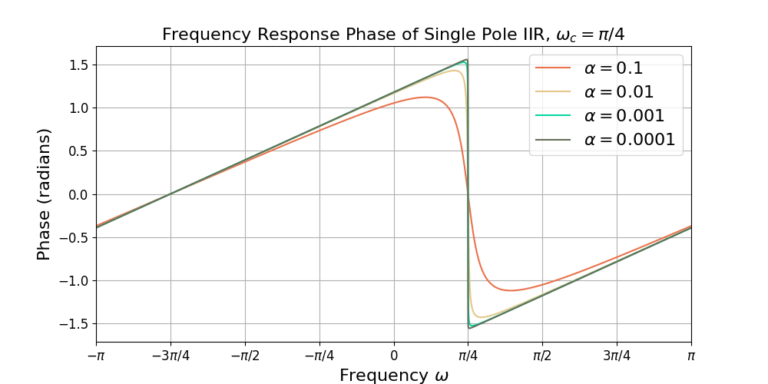# Wave Walker DSP

## DSP Algorithms for RF Systems

DSP for Beginners: Simple Explanations for Complex Numbers! The second edition includes a new chapter on complex sinusoids.

Band Pass Single Pole IIR Filter Design
February 1, 2023

#### Introduction

A low-pass single pole infinite impulse response (IIR) filter is transformed into a band pass filter using simple mathematics!

A previous blog described the frequency response of the single pole IIR filter and how it can be a substitute for a moving average filter.

Don’t miss these other blogs!

#### Reviewing the Low Pass Filter

A previous blog described how a specific implementation of a single pole IIR filter can be used to approximate a low pass moving average filter. The impulse response of this filter is defined as

(1)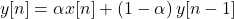which may also be referred to as an exponential moving averager or a leaky integrator. The impulse response in (1)

is simplified by setting

(2)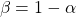such that

(3)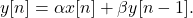The frequency response for the low pass filter (LPF) (3) is

(4)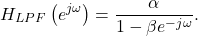#### Transformation into Band Pass Filter

The LPF single pole IIR filter can be transformed into a band pass filter (BPF) through complex frequency shifting. The center frequency of the band pass filter is defined to be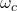and the frequency is shifted according to the substitution

(5)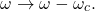The frequency response for the BPF filter is therefore

(6)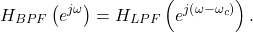The LPF frequency response (4) is substituted into (6),

(7)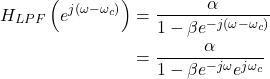The frequency response is represented using the output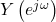and the input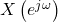(8)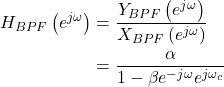which is rearranged into

(9)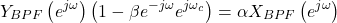and simplified as

(10)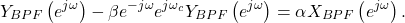The impulse response is obtained by applying the inverse Z-transform

(11)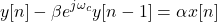which is more commonly written as

(12)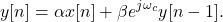The feedback filter coefficient for the LPF is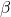however the bandpass version includes a complex weight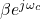.

#### Plotting Examples

The following plots show the frequency response for the band pass single pole IIR with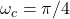. Figure 1 gives the magnitude of the frequency response and Figure 2 gives the phase of the frequency response. Compare the plots against the LPF in the previous blog post.